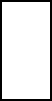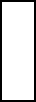Disclaimer: This is an example of a student written essay.

Any opinions, findings, conclusions or recommendations expressed in this material are those of the authors and do not necessarily reflect the views of UKEssays.com.

# Measuring Processes of Pipelining

 ✅ Paper Type: Free Essay ✅ Subject: Computer Science ✅ Wordcount: 2056 words ✅ Published: 12th Mar 2018

• Sakshi Dua

Abstract

Discuss the method to measure the performance of pipelining. Give a space-time diagram for visualizing the pipeline behavior for a four-stage pipeline. Also discuss some way to control a pipeline for collision free operations.

Introduction

Pipelining: A pipelining is a series of stages ,where some work is done at each stage .The work is not finished until it has passed through all stages.It is a technique used in advanced microprocessors where the microprocessor begin executing a second instruction before the first has been completed

Three performance measures pipeline are provided:-

1. Speed-up S(n)
2. Throughput U(n)
3. Efficiency E(n) .

Speedup S(n):-

Consider the execution of m tasks (instructions) using n-stages (units) pipeline.

n+m-1 time units are required to complete m tasks.

it is assumed that the unit time T = t units.

Speed-up S(n) = Time using sequential processing

————————————-

Time using pipeline processing

= m * n * t

————

(n + m – 1)* t

= m * n

——–

n + m -1

Lim S(n) = n

m→∞

i.e. n – fold increase in speed is theoretically possible.

Throughtput T(n):-

Throughtput U(n)= # of task executed per unit time

= m

————-

(n + m – 1)* t

Lim U(n) = 1

m→∞

Efficiency E(n):-

Efficiency E(n) = Ratio of the actual speed-up to maximum speed-up

= speed-up

———-

n

= m

————

n + m -1

Lim E(n) = 1

m→∞

Space Time Diagram For Four Stage Pipeline

The behavior of pipeline can be illustrated with space time diagram that the segment or stage utilization as a function of time .The horizontal axis displays the time in clock cycles and the vertical axis gives the segment number.The Diagram shows 6 tasks T1 through T6 executed in 4 segments.

Task T1 is handled by segment 1.after the first clock,segment 2 is busy with T1,while segment is busy with task T2.Continuing in this manner,the first task T1 is completed after the fourth clock cycle.From then on,the pipe completes a task every clock cycle.clockI/ps

S1 R1 S2 R2 S3 R3 S4 R4

DIAGRAM: FOUR STAGE PIPELINE

 1 2 3 4 5 6 7 8 9 T1 T2 T3 T4 T5 T6T1 T2 T3 T4 T5 T6 T1 T2 T3 T4 T5 T6 T1 T2 T3 T4 T5 T6

clock

Stage:1

2

3

4

SPACE TIME DIAGRAM FOR PIPELINE

For example:-

Consider the case where n- stages pipeline with a clock cycle time tp is used to execute m tasks. The first task t1 requires a time equal to ntp to complete its operation since there are n stages in the pipe. The remaining m-1 task emerge from the pipe at the rate of one task per clock cycle and they will be completed after a time equal to (m-1)tp. Therefore, to complete m tasks using a n-stages pipeline requires n+(m-1) clock cycles.

For eg. Above diagram shows four stages and 6 tasks. The time required to complete all the operations is

4+(6-1)=9 clock cycles.

Consider a non pipeline unit that performs the same operation and takes a time equal to tn to complete each task. The total time required m tasks is mtn. The speedup of a pipeline processing over a equivalent non pipeline processing is defined by the ratio

S(n)= mtn

———

(n+m-1)tp

As the no. Of tasks increases , m becomes much larger than m-1 and n+m-1 approaches the value of m. Under the condition , the speedup becomes

S(n)= tn

—–

tp

Assume that the time it takes to process a task is the same in the pipeline and non pipeline circuits, we will have tn = ntp including this assumption, the speedup reduces to

S(n)= ntp

——= N

tp

This shows that the theoretical max. Speedup that a pipeline can provide is n,where n is the no. Of stagessegments in the pipeline.

To clarify the meaning of the speedup ratio, let the time it takes to process a suboperation in each segment be equal to

tp=20 ns

Assume that the pipeline has n stages and executes n =100 tasks in sequence. The pipeline system will take (n+m-1)tp

=(4+99)*20

=2060 ns to complete.

Assuming that tn=mtp

4*20=80 ns,

A non pipeline system requires mntp=100*80=8000 ns to complete the 100 taks. The speedup ratio is equal to the

8000/2060=3.88.

As the no. Of tasks increases,the speedup will approach 4, which is equal to the no. Of stages in the pipeline.

If assume that

tn=60 ns,

the speedup becomes

60/20=3.

Some way to control a pipeline for collision free operations

To avoid the collision in data dependency operation are:

1. Hardware Interlocks

It is an interlock circuit that detects instructions whose source operands are destinations of instructions farther up in the pipeline. Detection of the situation causes the instruction whose source is not available to be delayed by enough clock cycles to resolve the collision. This way the program maintains the sequence by using hardware to insert the required delays.

1. Operand Forwarding

It uses special hardware to detect a collision and then avoid it by routing the data through special paths between pipeline stages. This method requires additional hardware paths through multiplexers as well as the circuit that detects the collision.

It solves the data collision problem to the compiler that translates the high level language into a machine language program. The compiler for such computers is designed to detect a data collision and reorder the instructions as necessary to delay the loading of the collisioned data by inserting no-operation instructions. This way is referred to as delayed load.

To avoid the collision in branch instructions operations are:

1. Prefetch Target Instruction

This is used to handling a conditional branch is to prefetch the target instruction in the additional to the instruction following branch. Bath are saved until the branch is executed. If the branch condition is successful, the pipeline continues from the branch target instruction. An extension the procedure is to continue fetching instructions from both places until the branch decision is of the correct program flow.

1. Branch Target Buffer

The BTB is an associative memory included in the fetch segment of the pipeline. Each entry in the BTB consists of the address of a previously executed branch instruction and the target instruction for that branch. It stores the new few instructions after the associative memory BTB for the address of the instruction . If it is in the BTB,the instruction is available directly and prefetch continues from the new path. If the instruction is not in the BTB, the pipeline shifts to a new instruction stream and stores the target instruction in the BTB. Advantage is that branch instruction occurred previously are readily available in the pipeline without interruption.

A Variation of the BTB is the load buffer. This is a small very high speed register file maintained by instruction fetch segment of the pipeline. When a program loop is detected in the program, it is stored in the loop buffer in its entirely, including all branches. The program loop can be executed directly without having to access memory until he loop mode is removed by final branching out.

1. Branch Prediction

A pipeline with branch prediction uses some additional logic to guess he outcome of a conditional branch instruction before it is executed . The pipeline then begins

refetching the instruction stream from the predicted path. A correct prediction eliminates the wasted time caused by branch penalties.

1. Delayed Branch

This is the way to employed in RICS processors is the delayed branch. In the procedure, the compiler detects the branch instruction and instruction hat keep the pipeline operating without interruptions. An example of delayed branch is the insertion of a no operation instruction after a branch instruction . This causes the computer to fetch the target instruction during the execution of the no-operation instruction ,allowing a continuous flow of the pipeline.

View all

## DMCA / Removal Request

If you are the original writer of this essay and no longer wish to have your work published on UKEssays.com then please:

Related Services

Prices from

KD47

Approximate costs for:

• 1000 words
• 7 day deliveryHumanity University

Dedicated to your worth and value as a human being!

Related Lectures